# Boat in Current

A boat in current is a good example of relative velocity.### Calculation

Index

Relative velocity

 HyperPhysics***** Mechanics R Nave
Go Back

# Boat in Current: Resultant Speed and Bearing

 Boat in current as example of relative velocity. Assume the rower heads straight across the river and is carried downstream by the current.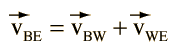Since the velocities form a right triangle, any velocity can be found from the triangle relationships if the other two are known.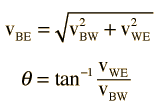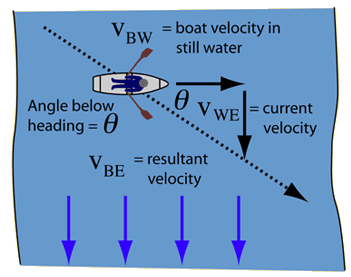Velocity unit
 If the rowing speed is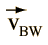=
 and the current speed is=
 then the resultant speed is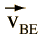= at an angle θ = ° downstream
 Alternate calculations: Rowing speed Speed of current
Index

Relative velocity

 HyperPhysics***** Mechanics R Nave
Go Back